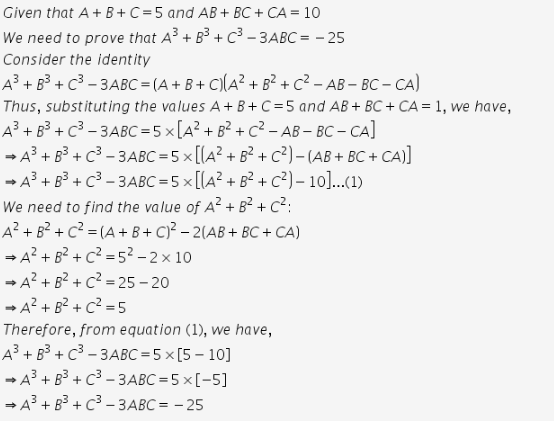# If A+B+C=5 and AB+BC+CA=10 then prove that A^3+B^3+C^3 -3ABC =-25

If A+B+C=5 and AB+BC+CA=10 then prove that A3+B3+C3 -3ABC =-25